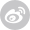【缠友园地】 缠论哲学和反身性哲学的思考，希望大家一起讨论一下
12345浏览 / 10个回复y=f(x)

x=F(y)

y=f(F(y))

x=F(f(x))52君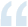y=f(x)

x=F(y)

y=f(F(y))

x=F(f(x))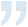【论坛公告】：
为构建一个安全健康的上网环境，打造文明规范的学习氛围，贯彻网络安全部门的相关政策，现特对社区发言进行相关整改。近期社区内用户发言将会进行多次审核，发言延后展现情况属于正常现象，如有其它问题可联系在线客服。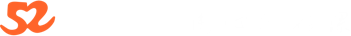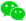使用微信登录